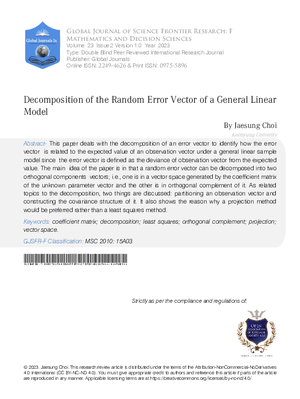### Decomposition of the Random Error Vector of a General Linear Model

Authors

• Jaesung Choi

Keywords:

coefficient matrix; decomposition; least squares; orthogonal complement; projection; vector space

Abstract

This paper deals with the decomposition of an error vector to identify how the error vector is related to the expected value of an observation vector under a general linear sample model since the error vector is defined as the deviance of observation vector from the expected value The main idea of the paper is in that a random error vector can be decomposed into two orthogonal components vectors i e one is in a vector space generated by the coefficient matrix of the unknown parameter vector and the other is in orthogonal complement of it As related topics to the decomposition two things are discussed partitioning an observation vector and constructing the covariance structure of it It also shows the reason why a projection method would be preferred rather than a least squares method

How to Cite

Jaesung Choi. (2023). Decomposition of the Random Error Vector of a General Linear Model. Global Journal of Science Frontier Research, 23(F2), 31–37. Retrieved from https://journalofscience.org/index.php/GJSFR/article/view/102614Published

2023-04-13

Issue

Section

Articles